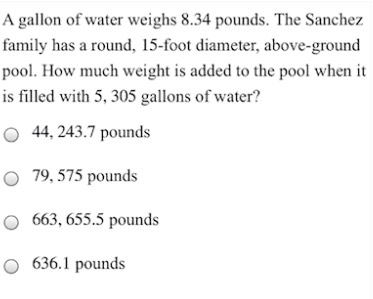### ¿Todavía tienes preguntas de matemáticas?

Pregunte a nuestros tutores expertos
Algebra
PreguntaA gallon of water weighs $$8.34$$ pounds. The Sanchez family has a round, $$15$$ -foot diameter, above-ground pool. How much weight is added to the pool when it is filled with $$5,305$$ gallons of water?

$$44,243.7$$ pounds

$$79,575$$ pounds

$$663,655.5$$ pounds

$$636.1$$ pounds